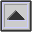SOUND INTENSITY

The sound energy transmitted per unit time through a unit area, thereby being a measure of the magnitude of a sound. The unit of measurement is the erg per second per square centimeter, or the watt per square meter. The THRESHOLD OF HEARING lies at 10-12 watts/m2, whereas the THRESHOLD OF PAIN is about 1 watt/m2.

The measurement of sound intensity is its INTENSITY LEVEL and is measured logarithmically in DECIBELs because of the wide range of intensities involved. See Appendix D for the conversion of intensity ratios to decibels.

Sound intensity is proportional to the square of the SOUND PRESSURE, which, being easier to measure, is more commonly used as a basis of sound measurement. Sound intensity in FREE FIELD situations varies inversely as the square of the distance from the sound source (see INVERSE-SQUARE LAW).

home